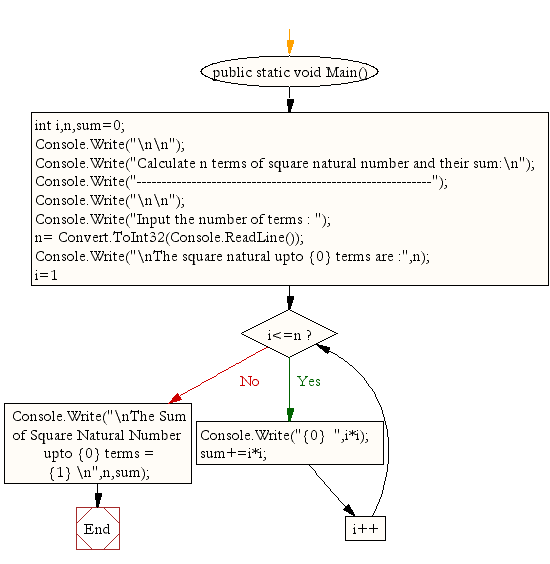﻿ C# - Calculate n terms of square natural number and sum# C# Sharp Exercises: Calculate n terms of square natural number and their sum

## C# Sharp For Loop: Exercise-25 with Solution

Write a program in C# Sharp to display the n terms of square natural number and their sum.
The series is as below:

1 4 9 16 ... n Terms

Sample Solution:-

C# Sharp Code:

``````using System;
public class Exercise25
{
public static void Main()
{
int i,n,sum=0;

Console.Write("\n\n");
Console.Write("Calculate n terms of square natural number and their sum:\n");
Console.Write("-----------------------------------------------------------");
Console.Write("\n\n");

Console.Write("Input the number of terms : ");
Console.Write("\nThe square natural upto {0} terms are :",n);
for(i=1;i<=n;i++)
{
Console.Write("{0}  ",i*i);
sum+=i*i;
}
Console.Write("\nThe Sum of Square Natural Number upto {0} terms = {1} \n",n,sum);
}
}
```
```

Sample Output:

```Calculate n terms of square natural number and their sum:
-----------------------------------------------------------
Input the number of terms : 10
The square natural upto 10 terms are :1  4  9  16  25  36  49  64  81  100
The Sum of Square Natural Number upto 10 terms = 385
```

Flowchart:C# Sharp Code Editor:

Contribute your code and comments through Disqus.

What is the difficulty level of this exercise?

Test your Programming skills with w3resource's quiz.

﻿